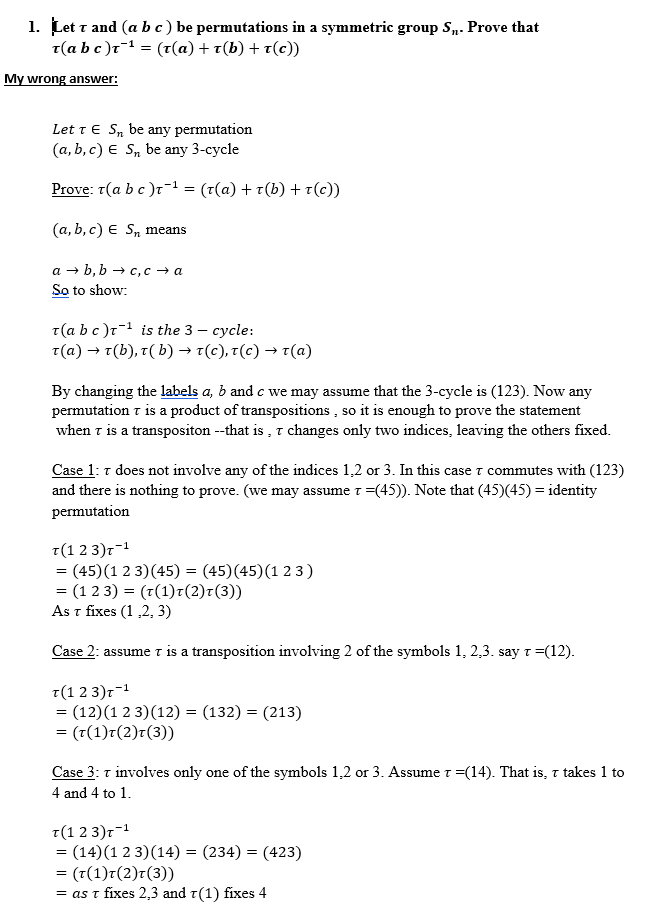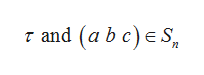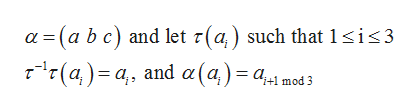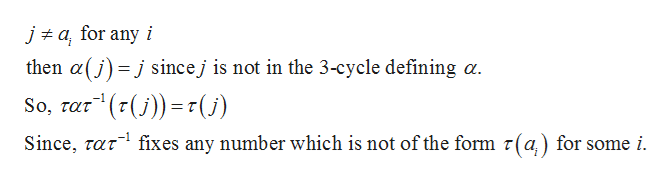1. Let T and (abc) be permutations in a symmetric group S. Prove thatτ (abc)τ1 = (τ(α) + τ(b) + τ(c)My wrong answerLet T E Sn be any permutation(a, b, c) E S be any 3-cycleProve: T(a b c)r-1 = (t(a) + T(b) r(c))(a, b, c) E Sn meansb, bc, c aSo to showа —aT(a b c)r is the 3 - cycle:τ (α) τ(b), τ ( b) > τ(c), τ(c) > τ(α)CBy changing the labels a, b and c we may assume that the 3-cycle is (123). Now anypermutation t is a product of transpositions, so it is enough to prove the statementwhen T is a transpositon --that is ,T changes only two indices, leaving the others fixedT.Case 1: T does not involve any of the indices 1,2 or 3. In this case T commutes with (123)and there is nothingr =(45)). Note that (45) (45) identityto prove. (we may assume TpermutationT(1 2 3)r= (45)(1 2 3)(45) = (45)(45)(1 2 3)= (1 2 3) (T(1)r(2)t(3))As t fixes (1,2, 3)-1Case 2: assumeT is a transposition involving 2 of the symbols 1, 2,3. say T (12)T(1 2 3)r= (12)(1 2 3) (12) (132) (213)(T(1)r (2)7(3))-1=Case 3: T involves onlyone of the symbols 1,2 or 3. Assume t -(14). That is, r takes 1 to4 and 4 to 1T(1 2 3)r(14) 1 2 3) (14) = (234) = (423)- (τ(1)τ (2)τ (3))= as t fixes 2,3 and t(1) fixes 4

Question

I need help understanding attached question for abstract algebra. My answer teacher said dosnt accountt for all numbers that may be in a set, i only used up to 5. Please help and show me correct wayhelp_outlineImage Transcriptionclose1. Let T and (abc) be permutations in a symmetric group S. Prove that τ (abc)τ1 = (τ(α) + τ(b) + τ(c) My wrong answer Let T E Sn be any permutation (a, b, c) E S be any 3-cycle Prove: T(a b c)r-1 = (t(a) + T(b) r(c)) (a, b, c) E Sn means b, bc, c a So to show а — a T(a b c)r is the 3 - cycle: τ (α) τ(b), τ ( b) > τ(c), τ(c) > τ(α) C By changing the labels a, b and c we may assume that the 3-cycle is (123). Now any permutation t is a product of transpositions, so it is enough to prove the statement when T is a transpositon --that is ,T changes only two indices, leaving the others fixed T. Case 1: T does not involve any of the indices 1,2 or 3. In this case T commutes with (123) and there is nothing r =(45)). Note that (45) (45) identity to prove. (we may assume T permutation T(1 2 3)r = (45)(1 2 3)(45) = (45)(45)(1 2 3) = (1 2 3) (T(1)r(2)t(3)) As t fixes (1,2, 3) -1 Case 2: assumeT is a transposition involving 2 of the symbols 1, 2,3. say T (12) T(1 2 3)r = (12)(1 2 3) (12) (132) (213) (T(1)r (2)7(3)) -1 = Case 3: T involves only one of the symbols 1,2 or 3. Assume t -(14). That is, r takes 1 to 4 and 4 to 1 T(1 2 3)r (14) 1 2 3) (14) = (234) = (423) - (τ(1)τ (2)τ (3)) = as t fixes 2,3 and t(1) fixes 4 fullscreen
Step 1

Given two permutations in a symmetric group arehelp_outlineImage Transcriptioncloser and (a b c) e S fullscreen
Step 2

Consider, the permutation of 3-cycle ashelp_outlineImage Transcriptionclosea (a b c) and let r(a) such that 1 sis3 r(a) a and a(a)= a1 md3 fullscreen
Step 3

Now take j such that j belo...help_outlineImage Transcriptioncloseja for any i then a(jjsincej is not in the 3-cycle defining a. ur"(r(j))= r(5) So, τατ Since, taT fixes any number which is not of the form r(a) for some i. fullscreen

Want to see the full answer?

See Solution

Want to see this answer and more?

Our solutions are written by experts, many with advanced degrees, and available 24/7

See Solution
Tagged in

Math